# TI-Nspire™ CX Premium Teacher Software

Visualize scientific concepts, facilitate discussions, create interactive classroom activities and more.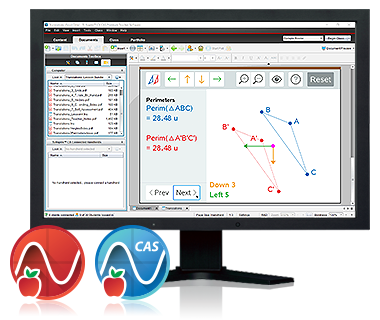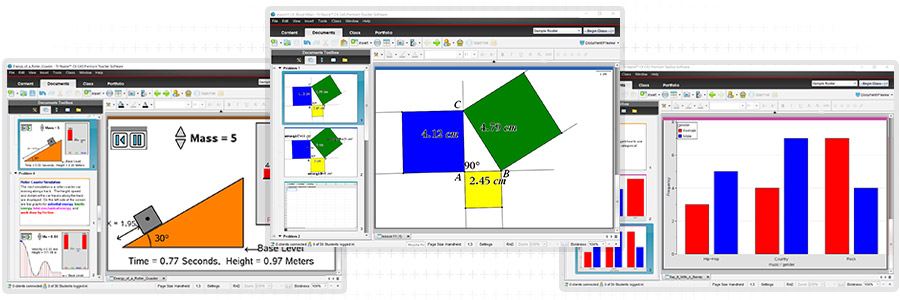## Open new paths to understanding

### New and faster interactive features motivate students to see and interact with math and science more deeply: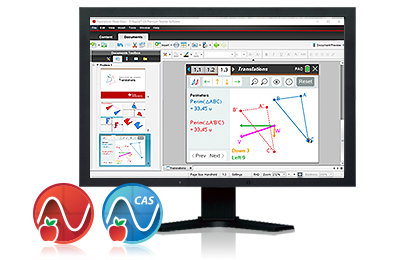• Animated path plot
Visualize function, parametric and polar graphs as they are drawn in real time.
• Dynamic coefficient values
Explore direct connections between dynamic coefficients in equations and graphs.
• Points by coordinates
Create dynamic points defined by coordinates, sliders or expressions quickly.
• Tick-mark labels
Label axes scales to create visual contexts that promote understanding.
• TI-Basic programming enhancements
Write code for visual illustration of key math, science and STEM ideas.
• Exact Math
With Exact Math, simplified expressions keep their mathematical structure and mathematical constants are recognized and simplified symbolically.

The CAS version of the TI-Nspire™ CX Premium Teacher Software enhancements also include:

• deSolve wizard
Reduce syntax errors in solving differential equations.
• Disable CAS
Disable algebraic functionality easily in the Press-to-Test dialog box or in document settings.

## Go beyond graphing

### The premium teacher software includes the same math, science and statistics applications that are built into TI-Nspire™ CX family graphing calculators.Calculator Perform computations and enter expressions, equations and formulas in proper math notation.Lists & Spreadsheet Perform mathematical operations on data and visualize the connections between the data and their plots.Graphs Plot and explore functions, equations and inequalities, animate points on objects and graphs, use sliders to explain their behavior and more.Data & Statistics Summarize and analyze data using different graphical methods such as histograms, box plots, bar and pie charts and more.Geometry Construct and explore geometric figures and create animations.Notes Enter notes, steps, instructions and other comments on the screen alongside the math.DataQuest™ Plug and play data collection and scientific analysis.ProgrammingAdd new functionality and create rich STEM activities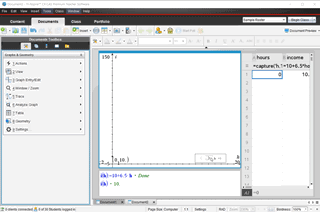#### Show the whole class, all at once

Instruct the students how to use their handhelds to solve the mathematical and scientific problems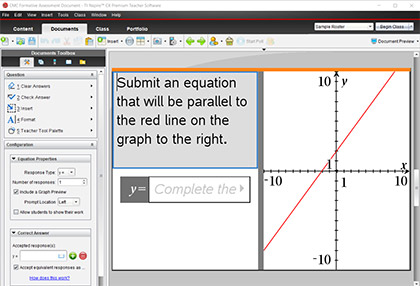#### Get the students talking about concepts

Encourage students to explain their work, defend their thinking and lead the class with Live Presenter.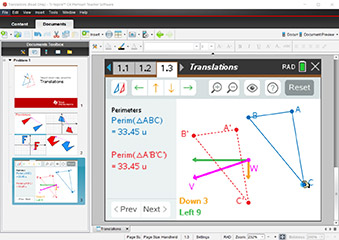#### Maximize learning time

Premium teacher software includes numeric and Computer Algebra System (CAS) functionality for maximum flexibility when demonstrating concepts, relationships and calculator usage.### Customer Support Center